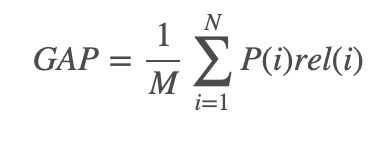Custom Callback Metric: GAP Score

(James Dietle) #1

For the Kaggle Landmark competition, they are using the GAP metric which fortunately already has code produced. But you can see the equation below.Trying to create a custom callback, we need the prediction, target and a probability/confidence. I think this works but would love a second pair of eyes.

``````#https://www.kaggle.com/c/landmark-recognition-2019/discussion/90752#latest-525286
# https://docs.fast.ai/callback.html

class GAP_vector(Callback):

''''
"Wrap a `func` in a callback for metrics computation."

Compute Global Average Precision (aka micro AP), the metric for the
This function takes predictions, labels and confidence scores as vectors.
In both predictions and ground-truth, use None/np.nan for "no label".

Args:
pred: vector of integer-coded predictions
conf: vector of probability or confidence scores for pred
true: vector of integer-coded labels for ground truth

Returns:
GAP score

Fastai Provides:
last_output: contains the last output spitted by the model (eventually updated by a callback)
last_loss: contains the last loss computed (eventually updated by a callback)
last_target: contains the last target that got through the model (eventually updated by a callback)
'''
_order=-20
def __init__(self):
nlearn:Learner
name:str='GAP_vector'

def on_epoch_begin(self, **kwargs):
# Creates empty list for predictions and targets
self.targs, self.preds,  self.loss = LongTensor([]), Tensor([]),Tensor([])

def on_batch_end(self, last_output:Tensor, last_target:Tensor, **kwargs):
# gets the predictions and targets for each batch

# Indicies are assoicated with class prediction
_, indices = torch.max(last_output, 1)
indices = torch.as_tensor(indices, dtype=torch.float)#, device=device)
# Finds the class with highest probability
last_output = F.softmax(last_output, dim=1)[:,-1]

# Appends the list with the predicted
self.preds = torch.cat((self.preds, indices.cpu()))
# Appends the list with the target
self.targs = torch.cat((self.targs, last_target.cpu().long()))
# Appends the list with the probability

self.loss = torch.cat((self.loss, last_output.cpu()))

def on_epoch_end(self, last_output, last_loss, last_metrics, **kwargs):
"Set the final result for GAP Score`."
# Creates the dataframe
x = pd.DataFrame({'pred': self.preds, 'conf': self.loss, 'true': self.targs})
# sorts the values by confidence
x.sort_values('conf', ascending=False, inplace=True, na_position='last')
# Makes a column for the number correct. Is true if the prediction is the same as target
x['correct'] = (x.true == x.pred).astype(int)
# creates column for predictions
x['prec_k'] = x.correct.cumsum() / (np.arange(len(x)) + 1)
# gets the total score
x['term'] = x.prec_k * x.correct
# divides by the count of true
gap = x.term.sum() / x.true.count()